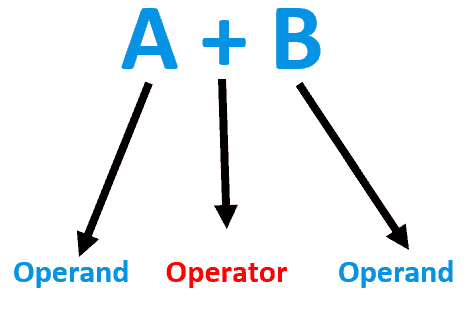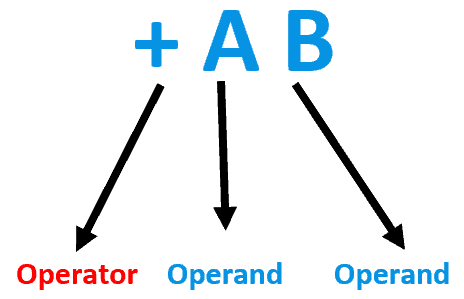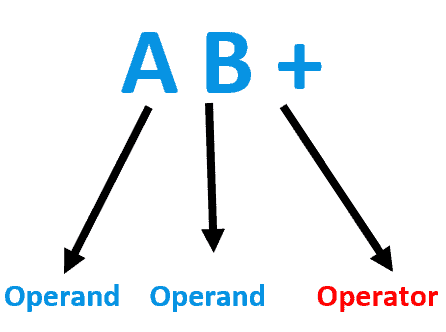## 1. Introduction

Mathematical formulas are often made more accessible by using parenthesis. However, in computers, expressions with multiple parentheses can be inefficient. So, mathematicians have created different notations such as infix, prefix, and postfix expressions to reduce computational work.

In this tutorial, we’ll explore these different ways of writing and evaluating expressions.

## 2. Infix Expressions

Infix expressions are the most usual type of expression. This notation is typically employed when writing arithmetic expressions by hand. Moreover, in the infix expression, we place the operator between the two operands it operates on.

For example, the operator “+” appears between the operands A and B in the expression “A + B”. The following figure depicts the example:Furthermore, infix expressions can also include parentheses to indicate the order of operations. In this way, we should observe the operator precedence rules and use parentheses to clarify the order of operations in expressions in infix notation.

Operator precedence rules specify the operator evaluation order in an expression. So, in an expression, operators with higher precedence are evaluated before operators with lower precedence.

Some operator precedence rules follow:

• Parentheses: expressions inside parentheses are evaluated first
• Exponentiation: exponents are evaluated next
• Multiplication and division: multiplication and division are evaluated before addition and subtraction

However, if an expression has multiple operators with the same precedence, the evaluation of those operators occurs from left to right.

## 3. Prefix Expressions

Prefix expressions, also known as Polish notation, place the operator before the operands.

For example, in the expression “+ A B”,  we place the “+” operator before the operands A and B, as demonstrated in the image next:We should consider that prefix expressions are evaluated from right to left. Thus, we apply each operator to its operands as it is encountered.

## 4. Postfix Expressions

Postfix expressions, also known as reverse Polish notation, where we place the operator after the operands.

For instance, in the expression “A B +”, the “+” we place the operator after the operands A and B. The figure next depicts the example:Hence, we can evaluate postfix expressions from left to right, with each operator being applied to its operands as encountered.

## 5. Comparison of the Expression Notations

The infix notation is the simplest notation for humans to read and write, but it requires more complex parsing algorithms for computers due to parentheses and operator precedence rules.

The prefix and postfix notations are computationally efficient and do not require parentheses or operator precedence tracking. Furthermore, the prefix notation can easily handle unary operators, while infix and postfix notations require special handling.

The infix notation uses parentheses for function arguments, while the prefix and postfix notations can use other delimiters.

The infix notation is the most usual notation for writing mathematical expressions, while the prefix and postfix notations are appropriate for particular applications. Examples of these applications are stack-based algorithms and programming languages.

## 6. Conversion of Infix to Postfix

One of the applications of postfix notation is to build a calculator or evaluate expressions in a programming language. In addition, we can evaluate postfix expressions efficiently using a stack data structure.

Therefore, postfix notation is effective for implementing algorithms such as postfix notation evaluation and expression parsing.

The process of converting an infix expression to a postfix expression involves the following steps:

1. First, we create an empty stack and an empty postfix expression
2. Next, we iterate through the infix expression from left to right and append operands to the postfix expression
3. If an operator is encountered, we pop operators from the stack and append them to the postfix expression until an operator with lower or equal precedence is found
4. The current operator is then pushed onto the stack
5. If a left parenthesis is encountered, we push it onto the stack
6. If a right parenthesis is encountered, we pop operators from the stack and append them to the postfix expression until a left parenthesis is found
7. Finally, we pop any remaining operators from the stack and append them to the postfix expression

Considering the previously defined steps, we can convert an infix expression like “5 + 6 * 2 – 3 / 2” into the postfix expression “5 6 2 * + 3 2 / -“. This notation facilitates a computer to evaluate the expression.

## 7. Conclusion

The infix, prefix, and postfix notations are three different ways of writing and evaluating expressions.

While infix expressions are common and intuitive for humans to read and write, prefix and postfix notations are computationally efficient and valuable for creating computer programs that manipulate expressions.

In particular, we can easily evaluate the postfix expressions with a stack data structure, making them appropriate for implementing some algorithms, such as parsers.